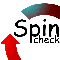Spinroot

A forum for Spin users

You are not logged in.

#1 2014-12-09 07:01:27

spinroot
forumRegistered: 2010-11-18
Posts: 678
Website

question about an unexpected result - and response

Posted here on behalf of [email protected]

--- from wh:
I would like to verify the impact of the time relationship between the use of resources in an access violation In the simulation program, the conflict can be seen the phenomenon does not exist.However, when model checking the LTL formula, the report displayed having two reachable states, I expected to zero.I do not know if I was wrong with formula or others.

Yours very respectfully, wang hong

1、promela program are as follows:

#define a_race  ( a_int1==WRITE && a_int2 == WRITE  || a_int1==READ && a_int2 == WRITE || a_int1==WRITE && a_int2 == READ )

mtype a_int1 = NULL, a_int2=NULL;
bool on_int1, on_int2;

proctype INT1() provided (on_int1 == true)
{
L: on_int2 = false;
a_int1 = WRITE;
a_int1 = NULL;
on_int2 = true;
goto L;
}
proctype INT2() provided (on_int2 == true)
{
L: on_int1 = false;
a_int2 = WRITE;
a_int2 = NULL;
on_int1 = true;
goto L;
}

init
{
on_int1 = true;
on_int2 = true;
run INT1();
run INT2();
}
ltl a_ltl { <> a_race }

-- ./pan -m2000 -N does not find an error.

---- response:
To verify the formula, you’ll have to run the ./pan executable with a –a flag (to catch liveness violations) and a –f flag (to secure weak fairness).
Doing so will give you a counter-example to the LTL formula.
The counter-example shows the following execution, which can continue forever, without ever making ‘a_race’ true:

\$ spin -t -p problem.pml
ltl a_ltl: <> (((((a_int1==WRITE)) && ((a_int2==WRITE))) || (((a_int1==READ)) && ((a_int2==WRITE)))) || (((a_int1==WRITE)) && ((a_int2==READ))))
starting claim 2
2:    proc  1 (INT2:1) problem.pml:20 (state 1)       [on_int1 = 0]
4:    proc  1 (INT2:1) problem.pml:21 (state 2)       [a_int2 = WRITE]
6:    proc  1 (INT2:1) problem.pml:22 (state 3)       [a_int2 = NULL]
8:    proc  1 (INT2:1) problem.pml:23 (state 4)       [on_int1 = 1]
10:    proc  0 (INT1:1) problem.pml:10 (state 1)       [on_int2 = 0]
12:    proc  0 (INT1:1) problem.pml:11 (state 2)       [a_int1 = READ]
14:    proc  0 (INT1:1) problem.pml:12 (state 3)       [a_int1 = WRITE]
<<<<<START OF CYCLE>>>>>
16:    proc  0 (INT1:1) problem.pml:13 (state 4)       [a_int1 = NULL]
18:    proc  0 (INT1:1) problem.pml:14 (state 5)       [on_int2 = 1]
20:    proc  1 (INT2:1) problem.pml:20 (state 1)       [on_int1 = 0]
22:    proc  1 (INT2:1) problem.pml:21 (state 2)       [a_int2 = WRITE]
24:    proc  1 (INT2:1) problem.pml:22 (state 3)       [a_int2 = NULL]
26:    proc  1 (INT2:1) problem.pml:23 (state 4)       [on_int1 = 1]
28:    proc  0 (INT1:1) problem.pml:10 (state 1)       [on_int2 = 0]
30:    proc  0 (INT1:1) problem.pml:11 (state 2)       [a_int1 = READ]
32:    proc  0 (INT1:1) problem.pml:12 (state 3)       [a_int1 = WRITE]
spin: trail ends after 32 steps
#processes: 2
a_int1 = WRITE
a_int2 = NULL
on_int1 = 1
on_int2 = 0

32:    proc  1 (INT2:1) problem.pml:20 (state 1)
32:    proc  0 (INT1:1) problem.pml:13 (state 4)
32:    proc  - (a_ltl:1) _spin_nvr.tmp:3 (state 3)
2 processes created

Offline